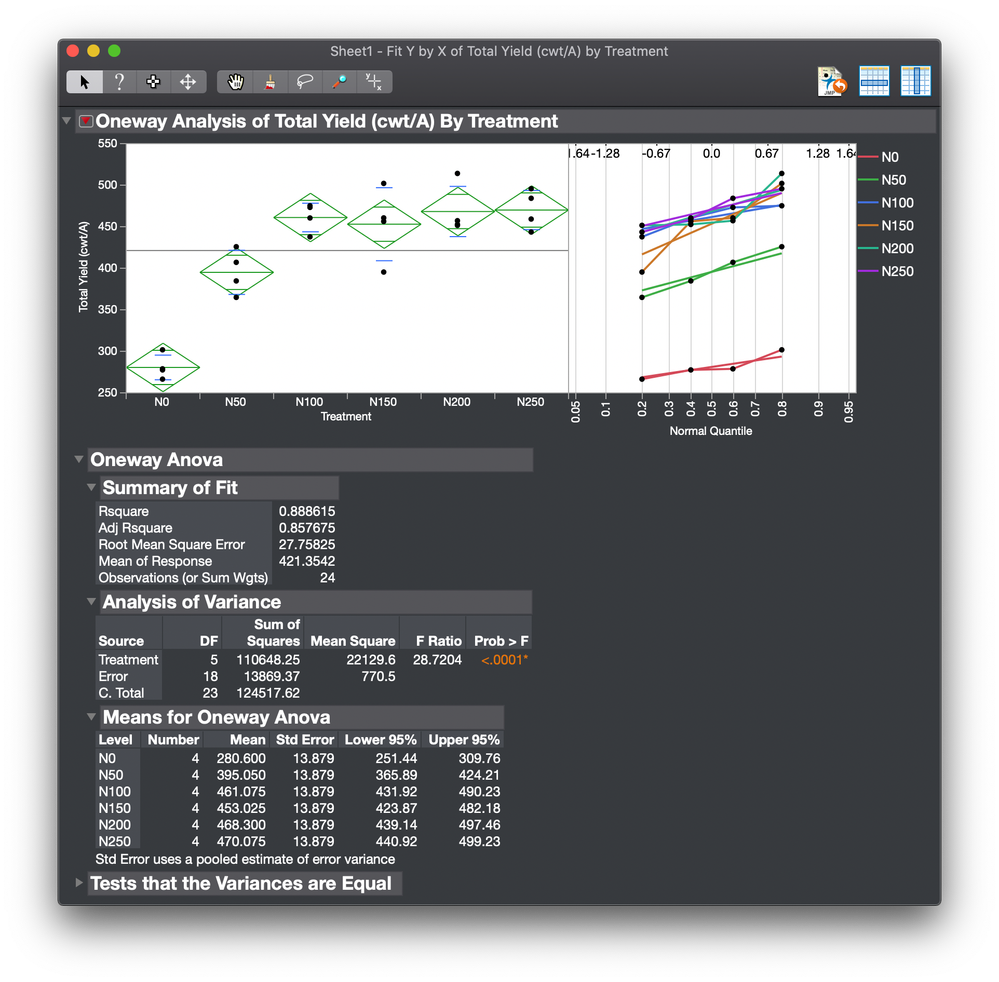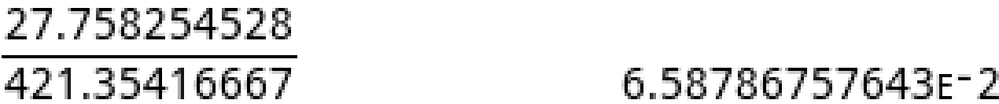Choose Language Hide Translation Bar
Highlighted

8 REPLIES 8
Highlighted

## Re: How to compute coefficient of variation (CV) when have used randomized complete block design with 5 reps using JMP. When i compute it using JMP table -summary it comes 17 while calculating it manually through using error mean sum of square it comes to 7.

I do not know how you might have used Table > Summary to estimate the CV. I do not know how you might have manually calculated the CV. I cannot assess either one of these results.

I assume that you want to estimate the CV of the response. The estimate as RMSE/mean would be the best estimate. The fixed effects have been accounted for in the model sum of squares leaving the random effect alone in the RMSE.

Here is the analysis of variance using the Oneway platform:So the estimated CV is about 7%:Learn it once, use it forever!
Highlighted

## Re: How to compute coefficient of variation (CV) when have used randomized complete block design with 5 reps using JMP. When i compute it using JMP table -summary it comes 17 while calculating it manually through using error mean sum of square it comes to 7.

Thank you so much

Another questions- Since I have used blocks/reps, why we not used reps in the model.

Also, should I use fit model or fit Y by X? I have analyzed the data and look the attached table and advise.

Highlighted

## Re: How to compute coefficient of variation (CV) when have used randomized complete block design with 5 reps using JMP. When i compute it using JMP table -summary it comes 17 while calculating it manually through using error mean sum of square it comes to 7.

I did not see any blocking structure in your data set. If you actually blocked the runs then you have to decide if their effect is fixed or random before adding to add a term to estimate this effect.

Do not enter replicate in the model. It is assumed to be the random error in the response. It is assumed to be normally distributed with constant variance. It is used to estimate the RMSE, a model-dependent estimate of the model errors. It should represent the normal variation run-to-run under complete randomization.

Both the Oneway platform and the Fit Least Squares platform can estimate the model parameters but they differ with respect to many available options. Also, if you decide to add a block effect, then you cannot use Oneway.

Learn it once, use it forever!
Highlighted

## Re: How to compute coefficient of variation (CV) when have used randomized complete block design with 5 reps using JMP. When i compute it using JMP table -summary it comes 17 while calculating it manually through using error mean sum of square it comes to 7.

You do not seem to understand the basics of linear statistical models or design of experiments from your use of terminology, for example, adding replicates as a term or referring to blocks and blocking. Your use of specific terms is confusing.

Where did you get the design? What effects were you trying to estimate? It seems as though you collected the data and then thought about how to analyze it. The optimal design is based on the intended model in the analysis.

Also, you did not state the purpose of your study. What are you trying to learn?

Learn it once, use it forever!
Highlighted

## Re: How to compute coefficient of variation (CV) when have used randomized complete block design with 5 reps using JMP. When i compute it using JMP table -summary it comes 17 while calculating it manually through using error mean sum of square it comes to 7.

Thank you. We have a lot of field variation. Therefore, the design used was randomized block design where blocks/reps were made against the field slop. The randomization of the treatments were done block wise. The blocks/reps were constructed to minimize the experimental error. Now, our main interest is to determine the treatment effect. So, should it be one way ANOVA or two way?

Highlighted

## Re: How to compute coefficient of variation (CV) when have used randomized complete block design with 5 reps using JMP. When i compute it using JMP table -summary it comes 17 while calculating it manually through using error mean sum of square it comes to 7.

If I understand what you say correctly, then you might have selected a RCBD, but you ran a split-plot design. So the replicate indicates the observations from the same field?

Learn it once, use it forever!
Highlighted

## Re: How to compute coefficient of variation (CV) when have used randomized complete block design with 5 reps using JMP. When i compute it using JMP table -summary it comes 17 while calculating it manually through using error mean sum of square it comes to 7.

You factor levels are labelled N0, N50, N100, N150, N200, and N250. This factor enters the design as a categorical factor with 6 levels. It is therefore modeled as a nominal effect. Is it, in fact, a continuous factor N that is tested at levels 0, 50, 100, 150, 200, and 250? That would change the modeling.

Your data was stored in an Excel workbook. Did you use JMP to design the experiment? I imported the worksheet. I added some useful column properties. I re-defined replicate as Whole Plot. I added it to the model as a random effect. (It is not a block, it is a restriction on randomization - you did not use a new field each time.) I fit two models. One uses the original treatment as a categorical factor. The other uses a new factor N as a continuous effect with a non-linear component.

I attached the new JMP data table.

Learn it once, use it forever!
Highlighted

## Re: How to compute coefficient of variation (CV) when have used randomized complete block design with 5 reps using JMP. When i compute it using JMP table -summary it comes 17 while calculating it manually through using error mean sum of square it comes to 7.

I have attached the field lay out, so, data generated by this field lay out should we use one way anova or two way?

Article Labels

There are no labels assigned to this post.